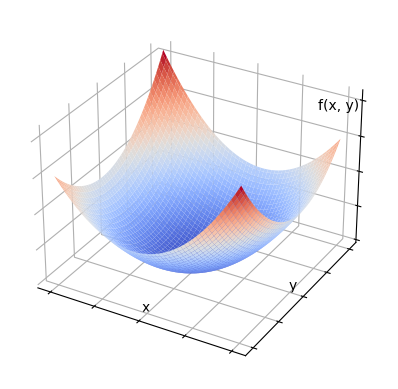# How to choose a solver¤

The best choice of solver depends a lot on your problem! If you're not too sure which to pick, here is some guidance for what usually works well.

Tip

For advanced users: you can often adjust the behaviour of individual solvers, e.g. by using alternative line searches. Moreover you can also mix-and-match components to derive custom optimisers, see here.

## Minimisation problems¤

For relatively "well-behaved" problems -- ones where the initial guess is likely quite close to the true minimum, and the function tends to look a bit like a bowl:then the best choice is usually an algorithm like optimistix.BFGS or optimistix.NonlinearCG. By assuming that your function is relatively well-behaved, then these try to take larger steps to get the minimum faster.

For "messier" problems -- where the surface of the function is not so well-behaved -- then a first-order gradient algorithm often works well. These work by taking many small steps, and never moving too far away from the current best-so-far. The Optax library is dedicated to such algorithms; as such you should try e.g. optimistix.OptaxMinimiser(optax.adabelief, learning_rate=1e-3, rtol=1e-8, atol=1e-8).

## Least-squares problems¤

These are an important special case of minimisation problems.

Either optimistix.LevenbergMarquardt or optimistix.Dogleg are recommended for most problems.

If your problem is particularly messy, as discussed above, then use a first-order gradient algorithm, e.g. optimistix.OptaxMinimiser(optax.adabelief(...), ...). These are compatible with optimistix.least_squares.

## Root-finding problems¤

For one-dimensional problems, use optimistix.Bisection.

For relatively "well-behaved" problems, then either optimistix.Newton or optimistix.Chord are recommended.

If your problem is a little bit messy, then try optimistix.LevenbergMarquardt or optimistix.Dogleg. (These are compatible with root-finding problems.)

If your problem is particularly messy, as discussed above, then use a first-order gradient algorithm, e.g. optimistix.OptaxMinimiser(optax.adabelief(...), ...). (These are also compatible with root-finding problems.)